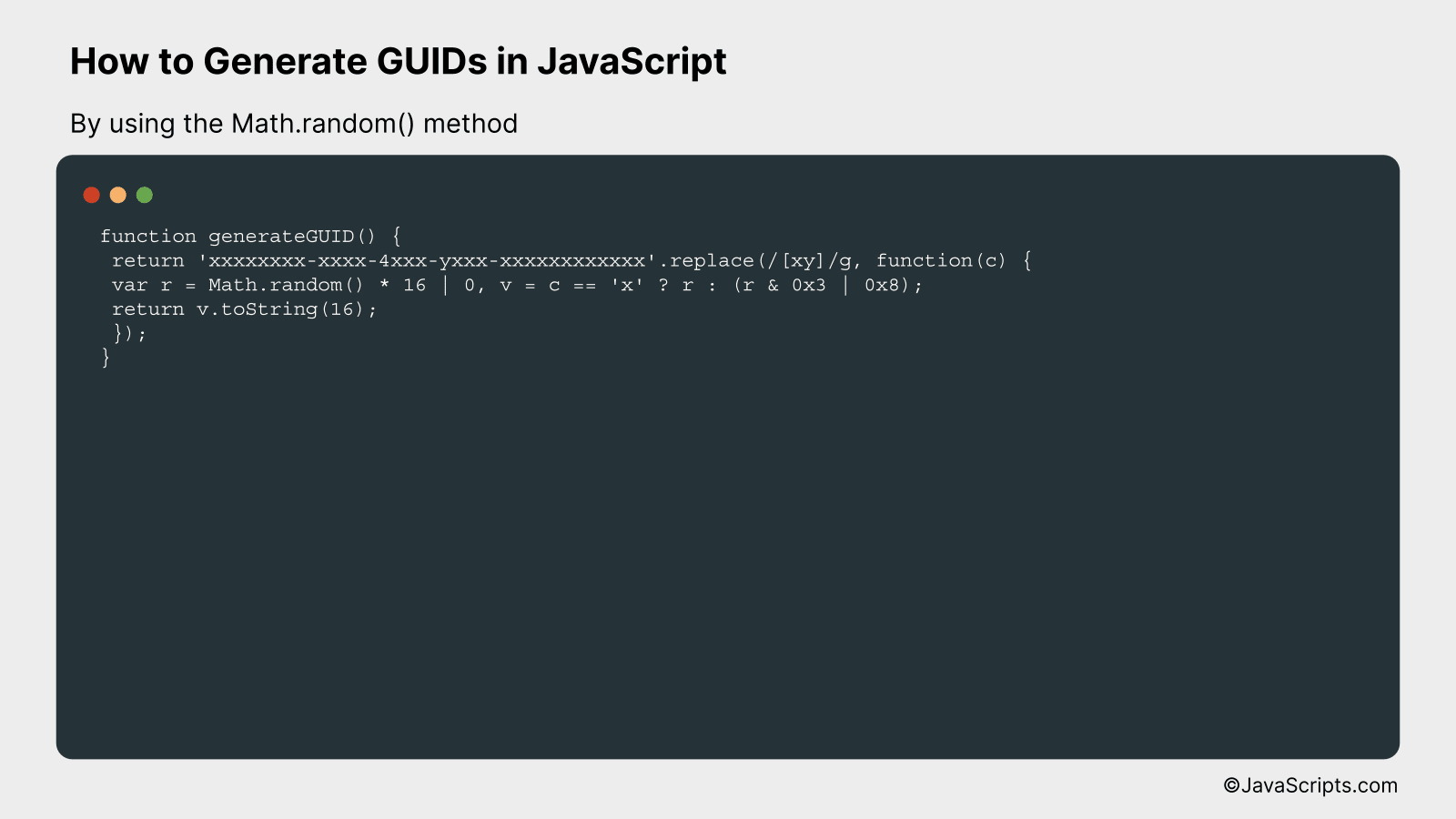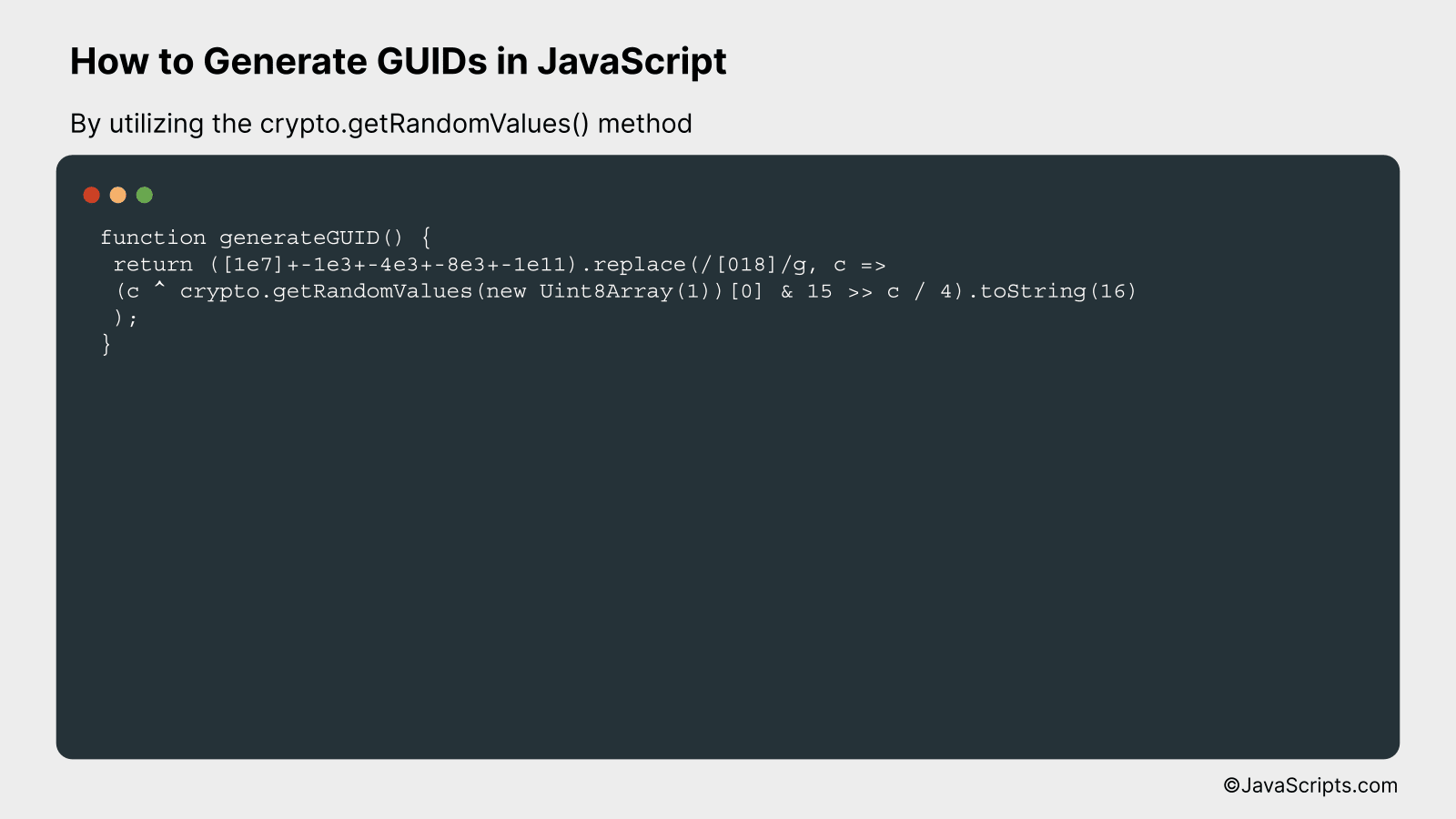# How to Generate GUIDs in JavaScript

There’s something uniquely satisfying about generating a unique identifier in the world of programming, isn’t there? A GUID (Globally Unique Identifier) is just that – a unique code that no one else in the world will have.

Now, you might be wondering how exactly to create a GUID in JavaScript. No worries, I’m here to guide you through it. Together, we’ll explore the straightforward process of generating these unique identifiers using accessible JavaScript methods.

In this post, we will be looking at the following 3 ways to generate GUIDs in JavaScript:

• By using the Math.random() method
• By utilizing the crypto.getRandomValues() method
• By leveraging third-party libraries like uuid

Let’s explore each…

## #1 – By using the Math.random() methodIn JavaScript, we can generate a GUID (Globally Unique Identifier) by exploiting the Math.random() function to generate random numbers, which we then convert to hexadecimal form and format to match the standard GUID format. An example of this would be generating a GUID like ‘3F2504E0-4F89-11D3-9A0C-0305E82C3301’.

``````
function generateGUID() {
return 'xxxxxxxx-xxxx-4xxx-yxxx-xxxxxxxxxxxx'.replace(/[xy]/g, function(c) {
var r = Math.random() * 16 | 0, v = c == 'x' ? r : (r & 0x3 | 0x8);
return v.toString(16);
});
}
```
```

### How it works

The provided function generates a GUID by replacing placeholder characters in a predefined GUID format string using JavaScript’s string replace() method with a callback function. This function generates random hexadecimal digits where required.

• Firstly, we create a template string ‘xxxxxxxx-xxxx-4xxx-yxxx-xxxxxxxxxxxx’ that corresponds to the standard GUID format.
• Then, the replace method is called on this string. We use a regular expression /[xy]/g to select all instances of ‘x’ and ‘y’ in the string.
• For each ‘x’ or ‘y’ found, the provided function is called. This function generates a random hexadecimal digit.
• The Math.random() method generates a random floating number between [0, 1), which is then multiplied by 16, giving us a random number in the range [0, 16).
• Using the bitwise OR operator with 0 (‘| 0’) ensures the result is a whole number by effectively discarding the fractional part.
• When the current character is ‘y’, we want to generate a number from [8, 11]. This is accomplished using the bitwise AND and OR operators with the random number.
• Finally, the number is converted to a hexadecimal string with the toString(16) method and replaces the original ‘x’ or ‘y’ character in the GUID format string.

## #2 – By utilizing the crypto.getRandomValues() methodThe following process generates GUIDs (Globally Unique Identifiers) in JavaScript by using the crypto.getRandomValues() method. It involves creating a function that returns a GUID by generating random hexadecimal values for each section of the identifier, in the standard GUID format.

``````
function generateGUID() {
return ([1e7]+-1e3+-4e3+-8e3+-1e11).replace(//g, c =>
(c ^ crypto.getRandomValues(new Uint8Array(1)) & 15 >> c / 4).toString(16)
);
}
```
```

### How it works

The above method generates a GUID using JavaScript’s cryptographic library’s getRandomValues method to generate random hexadecimal values. These values are then inserted into the structure of a standard GUID.

• The function begins by creating a template of a GUID format: “xxxxxxxx-xxxx-4xxx-yxxx-xxxxxxxxxxxx”. This is done by using the expression `[1e7]+-1e3+-4e3+-8e3+-1e11`, which actually presents this pattern.
• The `.replace` function is used to replace the characters in the GUID template. The regular expression `//g` matches all “0”, “1”, and “8” characters in the template.
• For each matched character, a callback function is run that generates a random hexadecimal value. This is where `crypto.getRandomValues(new Uint8Array(1))` comes into play. It generates a random number, which is then bit manipulated and converted to a hexadecimal string.
• The newly generated hexadecimal number replaces the matched character in the GUID template.
• Once all the “0”, “1”, and “8” characters have been replaced, the function returns the resulting string, which is a valid GUID.

## #3 – By leveraging third-party libraries like uuidThis will generate UUIDs (Universally Unique Identifiers) in JavaScript using the ‘uuid’ library, which will be demonstrated with a simple code example.

``````
const { v4: uuidv4 } = require('uuid');
let id = uuidv4();
console.log(id);
``````

### How it works

The above code uses the ‘uuid’ library in JavaScript to generate a UUID (Universally Unique Identifier). We import the version 4 function (v4) from ‘uuid’, call it to generate a unique id, and then log that id.

• First, we load the ‘uuid’ library. Specifically, we need the ‘v4’ function which generates random UUIDs. We use the ES6 destructuring assignment to import it: `const { v4: uuidv4 } = require('uuid');`
• Next, we call the ‘uuidv4’ function to generate a new UUID and store it in the ‘id’ variable: `let id = uuidv4();`
• Lastly, we log the generated UUID to the console: `console.log(id);`

Related:

In conclusion, generating GUIDs in JavaScript is a straightforward process. With a basic understanding of coding and the built-in Math random function, we can create unique identifiers for our web applications.

Remember, GUIDs are essential in providing each data entry with a unique tag, enhancing data management and security. So, keep practicing and make your JavaScript apps more robust and reliable.